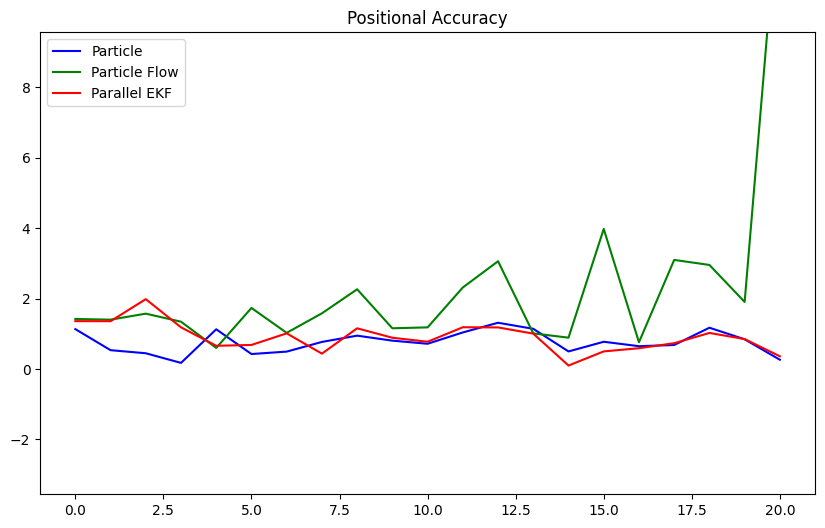# Gromov Particle Flow Filter¶

This example looks at utilising the Generalized Gromov method for stochastic particle flow filters.

## The Filter¶

We use the simple exact formula solution 1 to the equation:

$\frac{\partial p}{\partial \lambda} = -div(fp)+\frac{1}{2}div\left[Q(x)\frac{\partial p}{\partial x}\right]$

where $$p(x, λ)$$ is the conditional probability density of $$x$$ as a function of $$λ\in [0, 1]$$, $$f$$ is the drift function and $$Q$$ is the diffusion covariance.

Under the assumption that the prior density and likelihood are both multivariate Gaussian densities, and that $$Q$$ is a symmetric positive semi-definite matrix independent of $$x$$, we have the simple exact formula:

$Q = [P^{-1}+\lambda H^{T}R^{-1}H]^{-1}H^{T}R^{-1}H[P^{-1}+\lambda H^{T}R^{-1}H]^{-1}$

where $$Q$$ can be guaranteed to be positive, semi-definite by taking

$Q \rightarrow \frac{Q + Q^{T}}{2}$

Using $$Q$$, we can generate random samples of the diffusion for use in the stochastic flow of particles.

## Comparison¶

Comparing the bootstrap Stonesoup Particle filter, the Gromov particle flow filter, and the Gromov particle flow filter with parallel EKF covariance computation (2 using algorithm 2 with Gromov flow).

To note with particle flow, that resampling isn’t required and lower numbers of particles are needed, as it doesn’t suffer the same issues of degeneracy as bootstrap particle filter.

### One time-step¶

import datetime
import numpy as np
from scipy.stats import multivariate_normal

from stonesoup.types.groundtruth import GroundTruthPath, GroundTruthState
from stonesoup.models.measurement.nonlinear import CartesianToBearingRange
from stonesoup.types.detection import Detection
from stonesoup.predictor.particle import ParticlePredictor, ParticleFlowKalmanPredictor
from stonesoup.models.transition.linear import CombinedLinearGaussianTransitionModel, \
ConstantVelocity
from stonesoup.updater.particle import ParticleUpdater, GromovFlowParticleUpdater, \
GromovFlowKalmanParticleUpdater
from stonesoup.types.particle import Particle
from stonesoup.types.numeric import Probability
from stonesoup.types.state import ParticleState

np.random.seed(2020)

start_time = datetime.datetime(2020, 1, 1)
truth = GroundTruthPath([
GroundTruthState([4, 4, 4, 4], timestamp=start_time + datetime.timedelta(seconds=1))
])

measurement_model = CartesianToBearingRange(
ndim_state=4,
mapping=(0, 2),
noise_covar=np.diag([np.radians(0.5), 1])
)
measurement = Detection(measurement_model.function(truth.state, noise=True),
timestamp=truth.state.timestamp,
measurement_model=measurement_model)

transition_model = CombinedLinearGaussianTransitionModel([ConstantVelocity(0.05),
ConstantVelocity(0.05)])

p_predictor = ParticlePredictor(transition_model)
pfk_predictor = ParticleFlowKalmanPredictor(transition_model)  # By default, parallels EKF
predictors = [p_predictor, p_predictor, pfk_predictor]

p_updater = ParticleUpdater(measurement_model)
f_updater = GromovFlowParticleUpdater(measurement_model)
pfk_updater = GromovFlowKalmanParticleUpdater(measurement_model)  # By default, parallels EKF
updaters = [p_updater, f_updater, pfk_updater]

number_particles = 1000
samples = multivariate_normal.rvs(np.array([0, 1, 0, 1]),
np.diag([1.5, 0.5, 1.5, 0.5]),
size=number_particles)
# Note weights not used in particle flow, so value won't effect it.
weight = Probability(1/number_particles)
particles = [
Particle(sample.reshape(-1, 1), weight=weight) for sample in samples]


Run the filters

from matplotlib import pyplot as plt
from stonesoup.types.hypothesis import SingleHypothesis

fig = plt.figure(figsize=(10, 6))
ax = fig.add_subplot(1, 1, 1)
ax.axis('equal')

filters = ['Particle', 'Particle Flow', 'Parallel EKF']
particle_counts = [1000, 50, 50]
colours = ['blue', 'green', 'red']
handles, labels = [], []

for predictor, updater, colour, filter, particle_count \
in zip(predictors, updaters, colours, filters, particle_counts):
prior = ParticleState(particles[:particle_count], timestamp=start_time)

prediction = predictor.predict(prior, timestamp=measurement.timestamp)
hypothesis = SingleHypothesis(prediction, measurement)
post = updater.update(hypothesis)

data = np.array([particle.state_vector for particle in post.particles])
handles.append(ax.scatter(data[:, 0], data[:, 2], color=colour, s=2))

labels.append(filter)

ax.scatter(*truth.state_vector[[0, 2]], color='black')
ax.legend(handles=handles, labels=labels)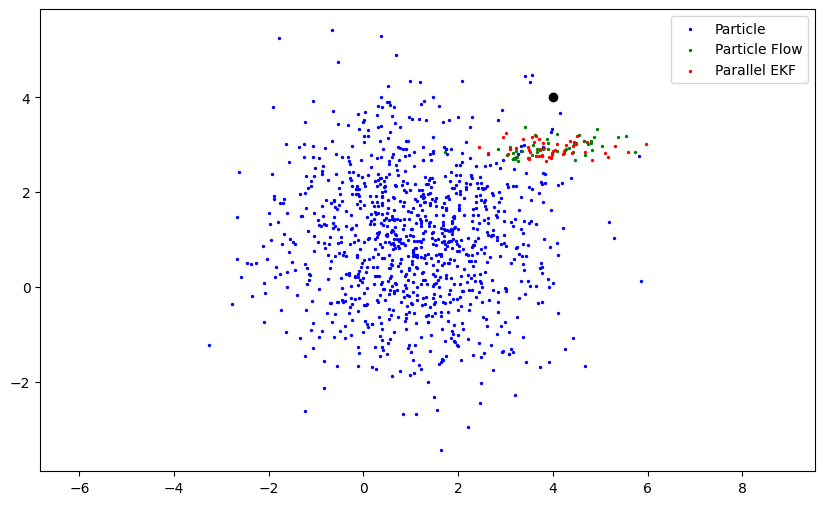### Multiple time-steps¶

truth = GroundTruthPath([GroundTruthState([0, 1, 0, 1], timestamp=start_time)])
for k in range(1, 21):
truth.append(
GroundTruthState(transition_model.function(truth[k-1],
noise=True,
time_interval=datetime.timedelta(seconds=1)),
timestamp=start_time+datetime.timedelta(seconds=k))
)

measurements = []
for state in truth:
measurement = measurement_model.function(state, noise=True)
measurements.append(Detection(measurement, timestamp=state.timestamp,
measurement_model=measurement_model))

number_particles = 1000
samples = multivariate_normal.rvs(np.array([0, 1, 0, 1]),
np.diag([1.5, 0.5, 1.5, 0.5]),
size=number_particles)
weight = Probability(1/number_particles)
particles = [Particle(sample.reshape(-1, 1), weight=weight)
for sample in samples]

from stonesoup.resampler.particle import SystematicResampler
from stonesoup.types.track import Track
from stonesoup.dataassociator.tracktotrack import TrackToTruth
from stonesoup.metricgenerator.manager import SimpleManager
from stonesoup.metricgenerator.tracktotruthmetrics import SIAPMetrics
from stonesoup.plotter import Plotter

updaters.resampler = SystematicResampler()  # Allow particle filter to re-sample

pa = dict()
siap_gen = SIAPMetrics(position_mapping=[0, 2])

for predictor, updater, colour, filter, particle_count \
in zip(predictors, updaters, colours, filters, particle_counts):
track = Track()
prior = ParticleState(particles[:particle_count], timestamp=start_time)

for measurement in measurements:
prediction = predictor.predict(prior, timestamp=measurement.timestamp)
hypothesis = SingleHypothesis(prediction, measurement)
post = updater.update(hypothesis)
track.append(post)
prior = track[-1]

plotter = Plotter()
plotter.plot_ground_truths(truth, [0, 2])
plotter.plot_measurements(measurements, [0, 2])
plotter.plot_tracks(track, [0, 2], particle=True, color=colour)
plotter.ax.set_title(filter)
plotter.ax.set_xlim(0, 30)
plotter.ax.set_ylim(0, 30)

metric_manager = SimpleManager(
[siap_gen], associator=TrackToTruth(association_threshold=np.inf))
metric_manager.add_data(tracks={track}, groundtruth_paths={truth})

pa[filter] = {metric for metric in metric_manager.generate_metrics()
if metric.title.startswith('time-based SIAP PA')}.pop()

•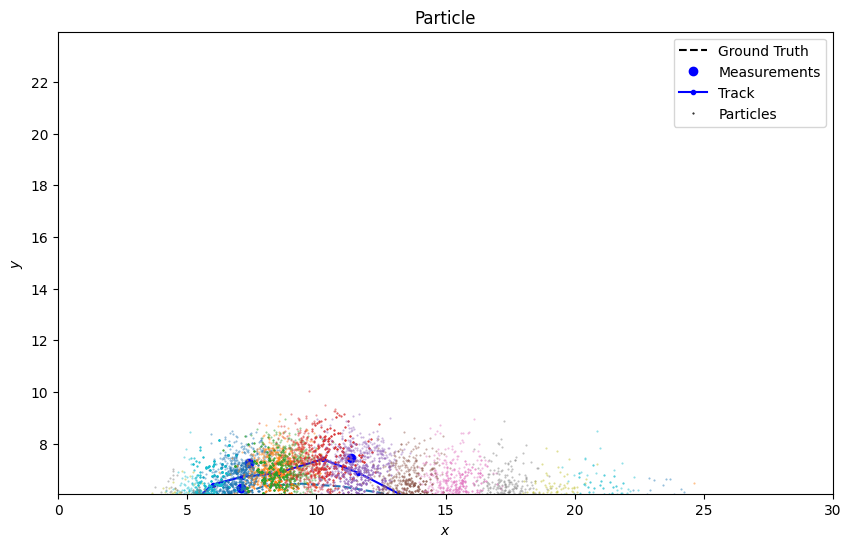•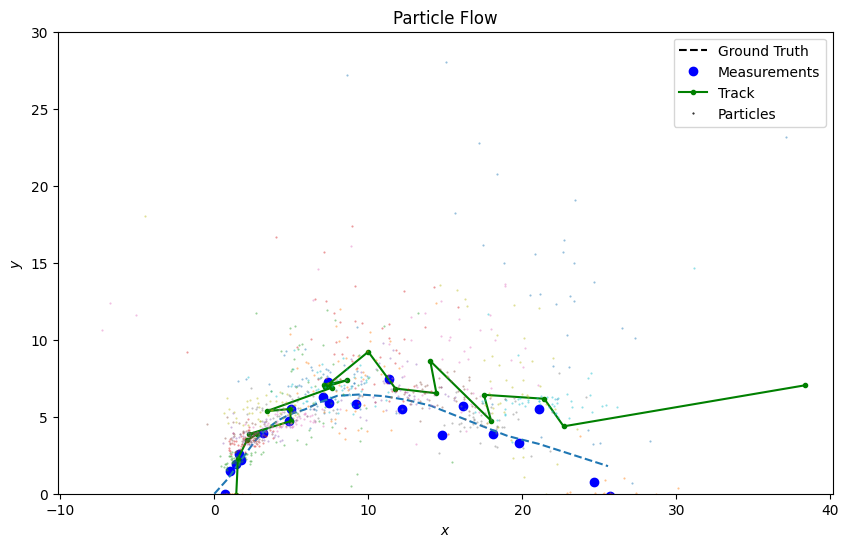•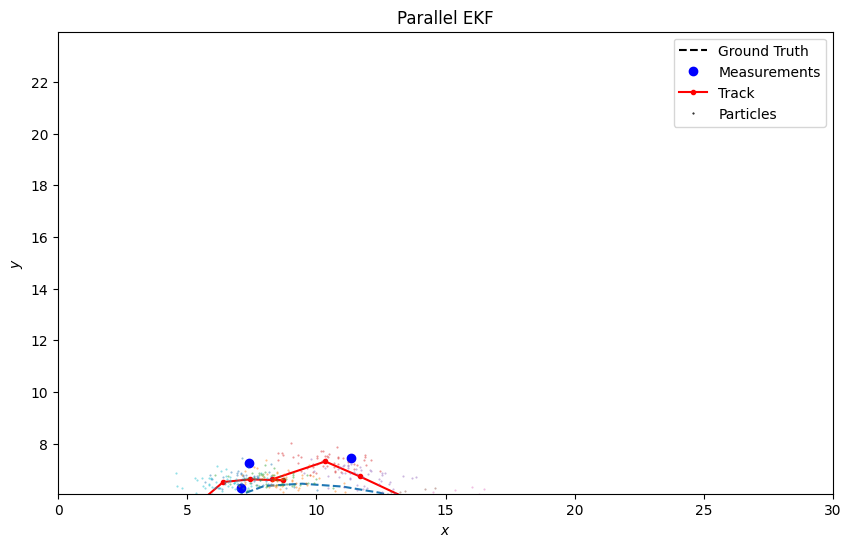### Positional Accuracy¶

from matplotlib.lines import Line2D

fig2 = plt.figure(figsize=(10, 6))
ax2 = fig2.add_subplot(1, 1, 1)
ax2.axis('equal')

handles = list()
labels = list()

for (filter, value), colour in zip(pa.items(), colours):
ax2.plot(range(len(value.value)), [elem.value for elem in value.value], color=colour)
handles.append(Line2D([], [], color=colour))
labels.append(filter)
ax2.legend(handles=handles, labels=labels)
ax2.set_ylim(0, 6)
_ = ax2.set_title('Positional Accuracy')